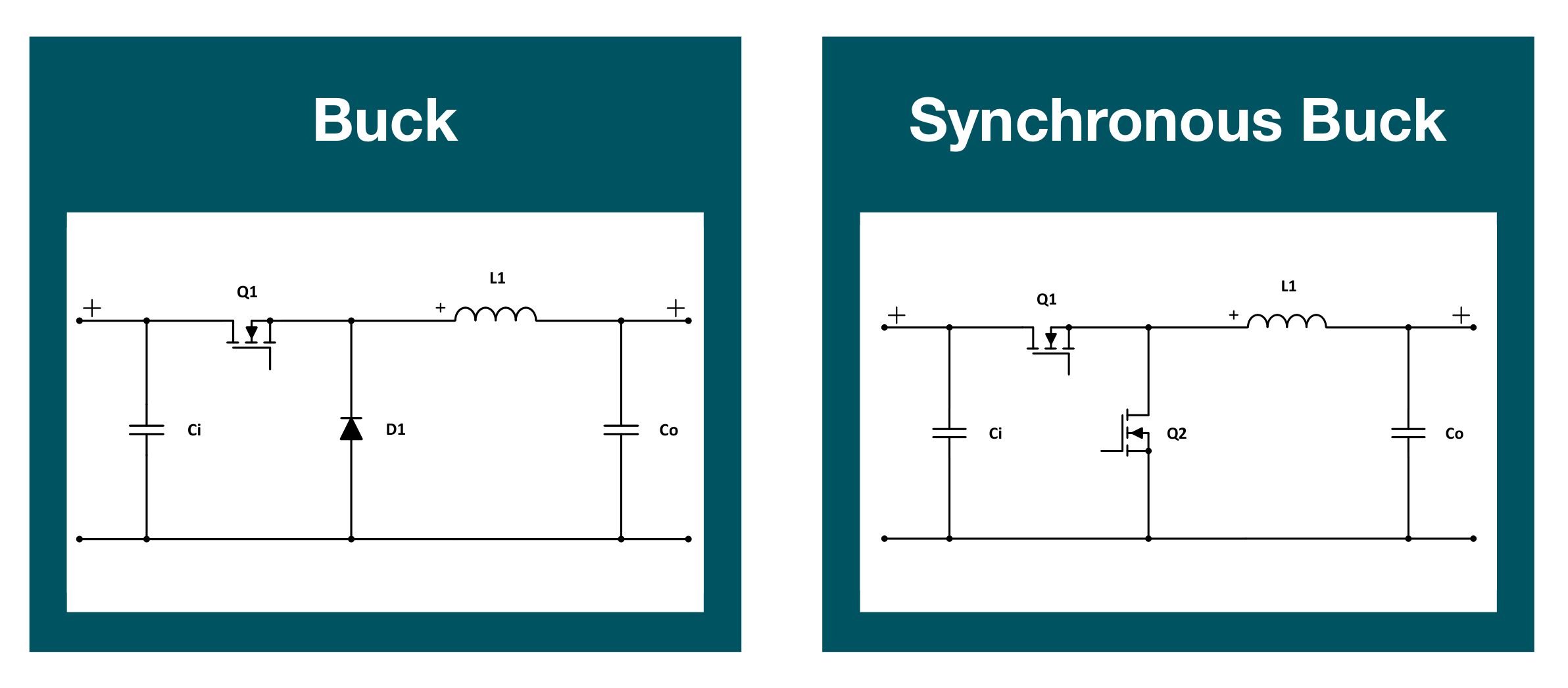# 电源拓扑 - 开关稳压（非隔离型）

## 开关稳压基础概念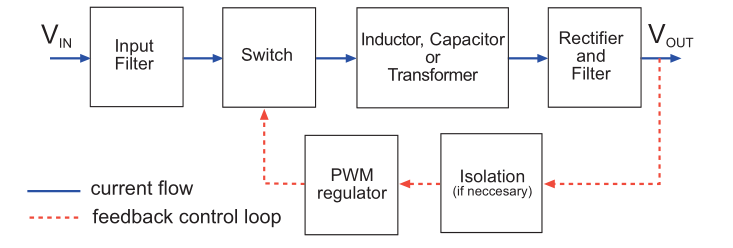• 一个完整周期的时间：$$T_{s}$$
• 电子开关接通的时间：$$T_{on}$$
• 占空比 $$\delta=\frac{T_{on}}{T_{s}}$$

## 开关稳压主要元件及特性

### 电感

1. 电感 $$L$$ 中有电流 $$I$$ 流过时，储存有 $$\frac{1}{2}LI^2$$ 的能量。
2. 电感 $$L$$ 两端电压 $$V$$ 不变时，因为 $$V=L\frac{di}{dt}$$，忽略内阻，电感电流的变化率就是 $$\frac{di}{dt}=\frac{V}{L}$$，即电感电流呈线性增加。
3. 正在储能的电感，如果突然切断原回路，电感会维持磁场不变，即电流不能瞬时突变，或者说伏·秒值不变。

$P(L)=\frac{LI^2f}{2}$

### 电容

$P(C)=\frac{CV^2f}{2}$

## 非隔离型 DC-DC 拓扑结构

### 降压型 Buck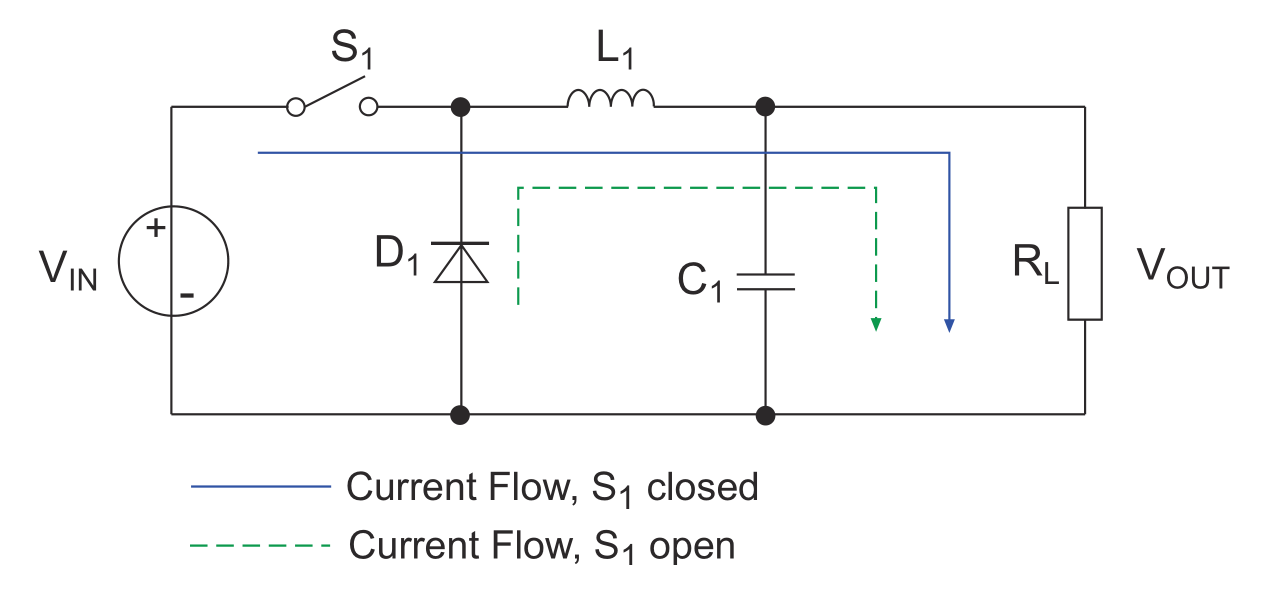1. 开关 $$S_1$$ 闭合（蓝色实线）
• 此时二极管 $$D_1$$ 截止，电流经过电感 $$L_1$$，给负载 $$R_L$$ 供电，同时将电能储存在电感 $$L_1$$ 和电容 $$C_1$$ 中。在电感线圈未饱和前，电流呈线性增加（电感内电流不能突变），输出电压不能立刻达到输入的电压值。
• 此时电感正向伏秒为：$$(V_{in}-V_{out})* T_{on}$$
2. 开关 $$S_1$$ 断开（绿色虚线）
• 电感 L 因自感惯性作用，其电流方向大小不能发生突变。这时候电流会流经由续流二极管 $$D_1$$ 提供的回路，继续为输出负载供电。此时电容 $$C_1$$ 也参与放电。
• 此时电感反向伏秒为：$$V_{out} * T_{off}$$

$V_{out}=\delta*V_{in}$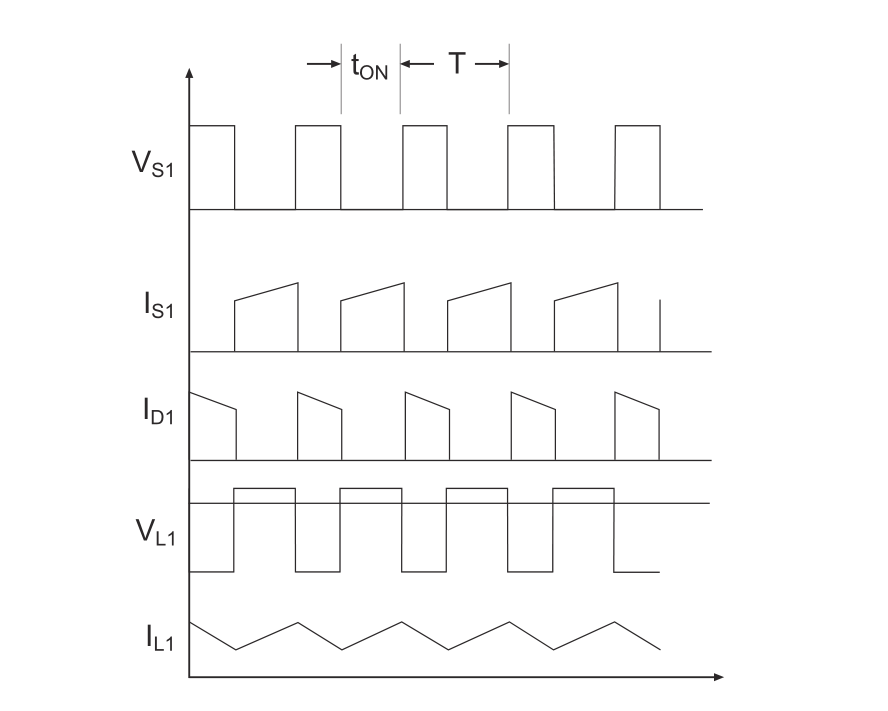### 升压型 Boost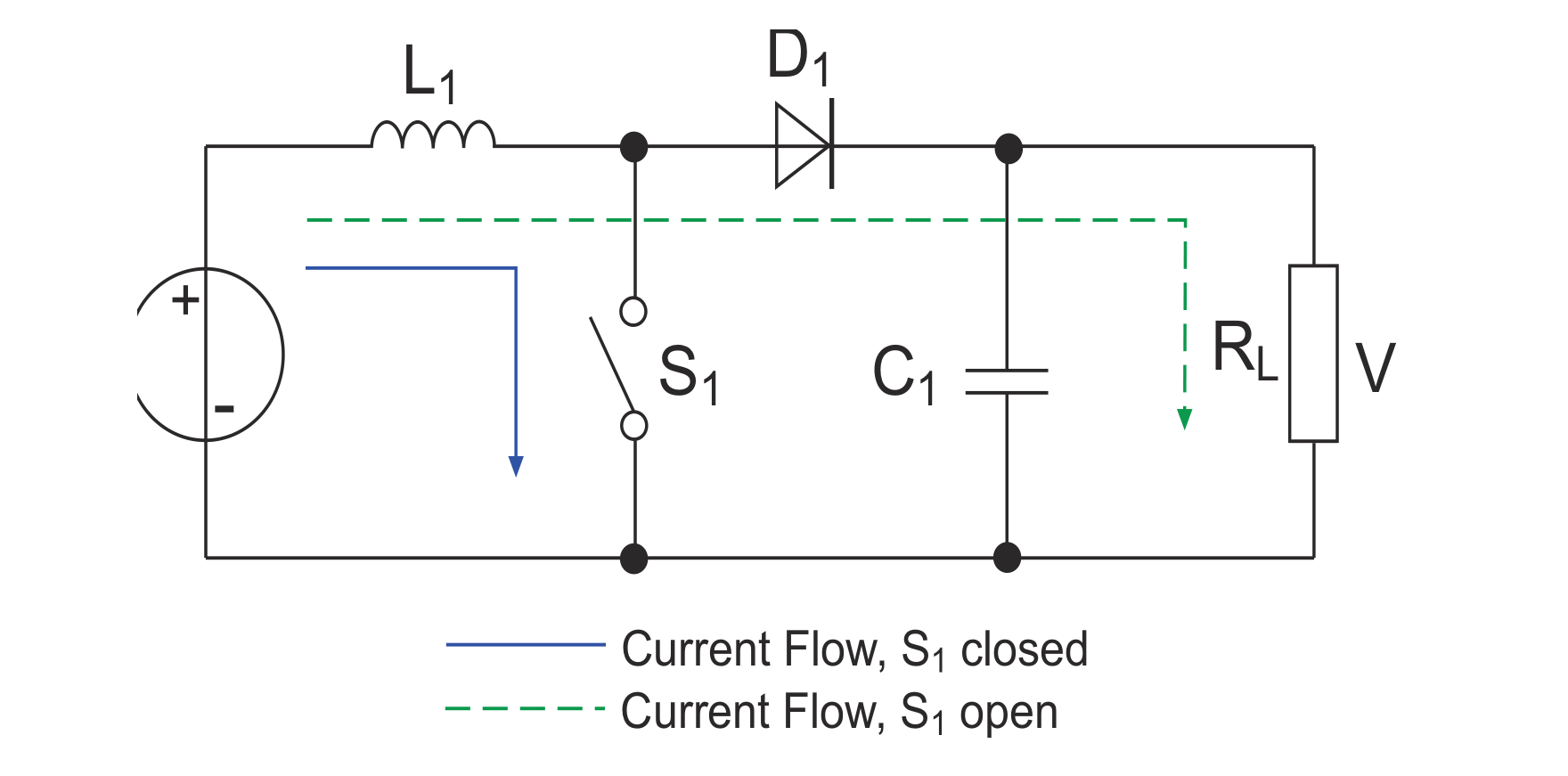1. 开关 $$S_1$$ 闭合（蓝色实线）
• 电流流经电感 $$L_1$$，在电感未饱和前，其电流以 $$\frac{V_{in}}{L_1}$$ 的速率线性增加，电能以磁能形式储存在电感中。此时电容 $$C_1$$ 为负载 $$R_L$$ 供电，假设此时负载两端电压为 $$V_0$$。因为开关 $$S_1$$ 闭合，所以二极管 $$D_1$$ 阳极接地，电容放出的电不能通过二极管。
• 此时电感正向伏秒为：$$V_{in}* T_{on}$$
2. 开关 $$S_1$$ 断开（绿色虚线）
• 由于电感 $$L_1$$ 上的电流不能突变，所以电感上的磁能转化为电压输出电流，以 $$\frac{V_{out}-V_{in}}{L_1}$$ 的速率线性下降，与电源 $$V_{in}$$ 形成串联，向电容 $$C_1$$ 和负载 $$R_L$$ 供电。当串联电压高于$$V_0$$ 时，电容 $$C_1$$ 状态为充电；当串联电压等于 $$V_0$$ 时，充电电流为零；当 $$V_0$$ 有下降趋势时，电容向负载放电，以维持 $$V_0$$ 不变。
• 此时电感反向伏秒为：$$(V_{out}-V_{in})* T_{off}$$

$V_{out}=\frac{\delta}{1-\delta}*V_{in}$

（当且仅当 V{in}<V 时成立）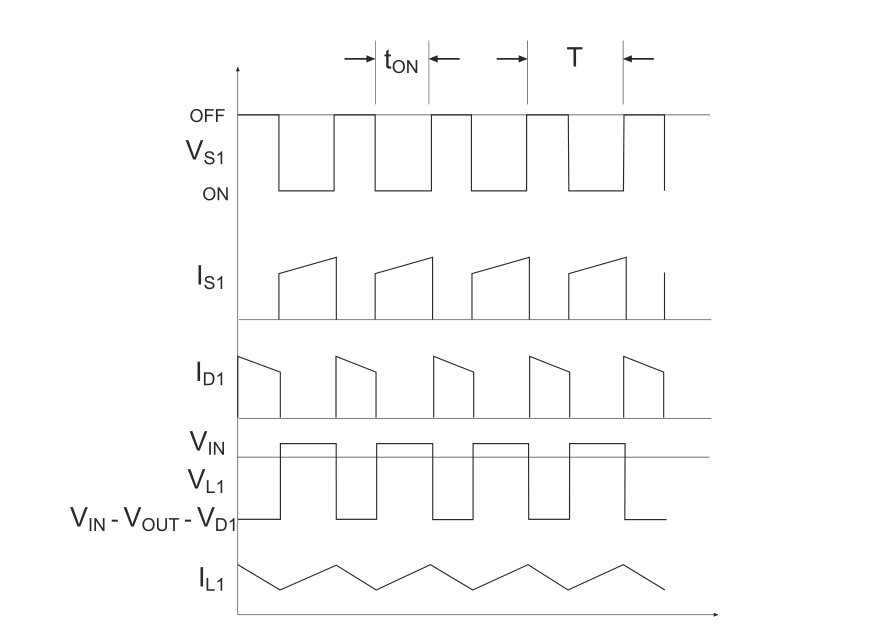### 升降压型 Buck-Boost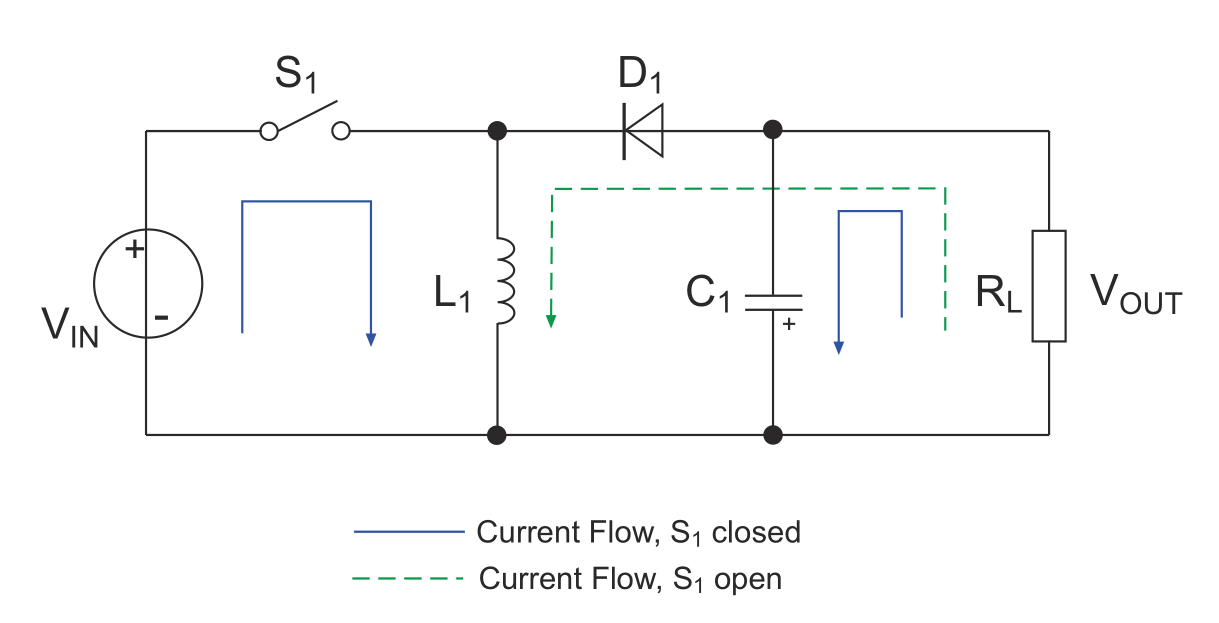1. 开关 $$S_1$$ 闭合（蓝色实线）
• 电流流经电感 $$L_1$$，在电感未饱和前，其电流以 $$\frac{V_{in}}{L_1}$$ 的速率线性增加；二极管 $$D_1$$ 反向截止，阻断从电源通向负载的电流。这段时间内存储在 $$C_1$$ 中的能量对负载供电。
• 此时电感正向伏秒为：$$V_{in}* T_{on}$$
2. 开关 $$S_1$$ 断开（绿色虚线）
• 由于电感 $$L_1$$ 上的电流不能突变，其电流以 $$\frac{V_{out}}{L_1}$$ 的速率线性下降，所以电流走绿色虚线回路，对负载供电，并给电容 $$C_1$$ 充电，二极管 $$D_1$$ 正向导通。流经电感的电流呈线性减小。由于电流的流向，输出电压对地而言是负值，所以这个拓扑结构的输出是反相的。
• 此时电感反向伏秒为：$$-V_{out}* T_{off}$$

$V_{out}=\frac{-\delta}{1-\delta}*V_{in}$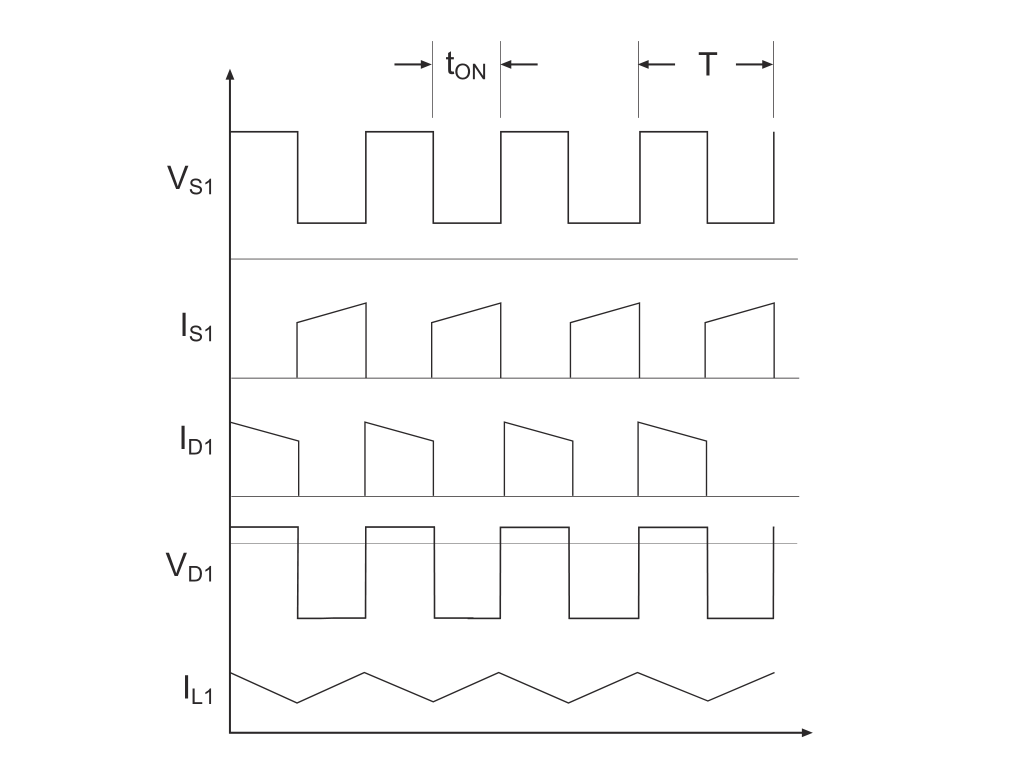## Buck 与 Boost 的连续与非连续模式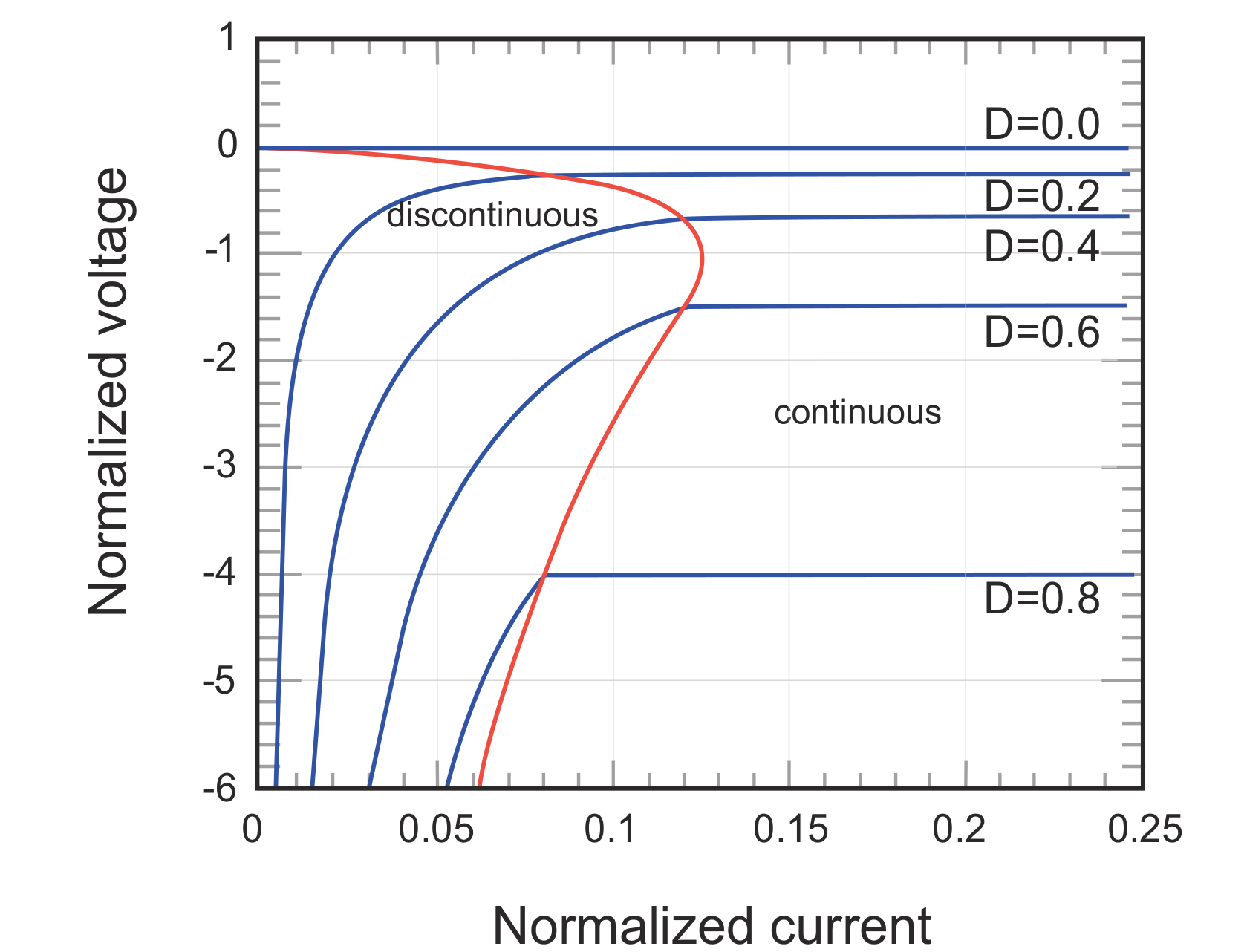## 同步与异步转换